# JP4672236B2 - Control device for synchronous motor - Google Patents

## Info

Publication number
JP4672236B2
JP4672236B2 JP2002565151A JP2002565151A JP4672236B2 JP 4672236 B2 JP4672236 B2 JP 4672236B2 JP 2002565151 A JP2002565151 A JP 2002565151A JP 2002565151 A JP2002565151 A JP 2002565151A JP 4672236 B2 JP4672236 B2 JP 4672236B2
Authority
JP
Japan
Prior art keywords
current
estimated
synchronous motor
axis
rotational speed
Prior art date
Legal status (The legal status is an assumption and is not a legal conclusion. Google has not performed a legal analysis and makes no representation as to the accuracy of the status listed.)
Active
Application number
JP2002565151A
Other languages
Japanese (ja)
Other versions
JPWO2002091558A1 (en
Inventor

Original Assignee

Priority date (The priority date is an assumption and is not a legal conclusion. Google has not performed a legal analysis and makes no representation as to the accuracy of the date listed.)
Filing date
Publication date
Application filed by 三菱電機株式会社 filed Critical 三菱電機株式会社
Priority to PCT/JP2001/003530 priority Critical patent/WO2002091558A1/en
Publication of JPWO2002091558A1 publication Critical patent/JPWO2002091558A1/en
Application granted granted Critical
Publication of JP4672236B2 publication Critical patent/JP4672236B2/en
Active legal-status Critical Current
Anticipated expiration legal-status Critical

• 230000001360 synchronised Effects 0.000 title claims description 104
• 244000171263 Ribes grossularia Species 0.000 claims description 110
• 230000003044 adaptive Effects 0.000 claims description 76
• 230000004907 flux Effects 0.000 claims description 73
• 239000002529 flux Substances 0.000 description 69
• 238000010586 diagrams Methods 0.000 description 22
• 238000004364 calculation methods Methods 0.000 description 18
• 239000011159 matrix materials Substances 0.000 description 16
• 230000014509 gene expression Effects 0.000 description 7
• 241001442055 Vipera berus Species 0.000 description 5
• 230000002542 deteriorative Effects 0.000 description 4
• 230000004044 response Effects 0.000 description 4
• 238000009795 derivation Methods 0.000 description 1
• 230000000694 effects Effects 0.000 description 1
• 239000000686 essences Substances 0.000 description 1
• 230000020169 heat generation Effects 0.000 description 1
• 230000004043 responsiveness Effects 0.000 description 1
• 230000001629 suppression Effects 0.000 description 1
• 238000004804 winding Methods 0.000 description 1

## Images

•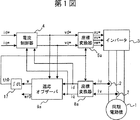•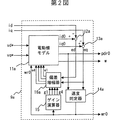•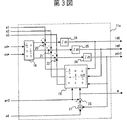•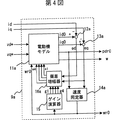•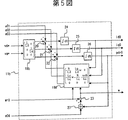•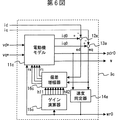•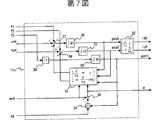•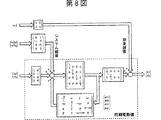•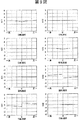•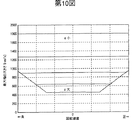•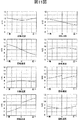•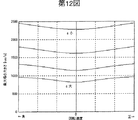•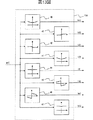•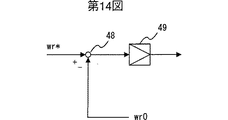•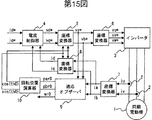•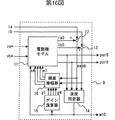## Classifications

• HELECTRICITY
• H02GENERATION; CONVERSION OR DISTRIBUTION OF ELECTRIC POWER
• H02PCONTROL OR REGULATION OF ELECTRIC MOTORS, ELECTRIC GENERATORS OR DYNAMO-ELECTRIC CONVERTERS; CONTROLLING TRANSFORMERS, REACTORS OR CHOKE COILS
• H02P6/00Arrangements for controlling synchronous motors or other dynamo-electric motors using electronic commutation dependent on the rotor position; Electronic commutators therefor
• H02P6/14Electronic commutators
• H02P6/16Circuit arrangements for detecting position
• H02P6/18Circuit arrangements for detecting position without separate position detecting elements
• HELECTRICITY
• H02GENERATION; CONVERSION OR DISTRIBUTION OF ELECTRIC POWER
• H02PCONTROL OR REGULATION OF ELECTRIC MOTORS, ELECTRIC GENERATORS OR DYNAMO-ELECTRIC CONVERTERS; CONTROLLING TRANSFORMERS, REACTORS OR CHOKE COILS
• H02P21/00Arrangements or methods for the control of electric machines by vector control, e.g. by control of field orientation
• H02P21/22Current control, e.g. using a current control loop
• HELECTRICITY
• H02GENERATION; CONVERSION OR DISTRIBUTION OF ELECTRIC POWER
• H02PCONTROL OR REGULATION OF ELECTRIC MOTORS, ELECTRIC GENERATORS OR DYNAMO-ELECTRIC CONVERTERS; CONTROLLING TRANSFORMERS, REACTORS OR CHOKE COILS
• H02P6/00Arrangements for controlling synchronous motors or other dynamo-electric motors using electronic commutation dependent on the rotor position; Electronic commutators therefor
• H02P6/14Electronic commutators

## Description

Technical field
The present invention relates to a control device that controls a synchronous motor without using a position sensor.
Background art
Generally, when controlling a synchronous motor, position sensors such as an encoder, a resolver, and a Hall element are required. However, the use of a position sensor in the control device for the synchronous motor is disadvantageous in terms of price, sensor reliability, wiring inconvenience, and the like. From such a viewpoint, a method for controlling a synchronous motor without using a position sensor has been proposed.
For example, a method for calculating the rotational position and rotational speed of a synchronous motor based on mechanical constants such as inertia, an induced voltage coefficient determined by magnet magnetic flux and the like, and electrical constants of the synchronous motor such as inductance and resistance There are inventions such as US Pat. No. 5,296,793, US Pat. No. 5,296,794, Japanese Patent Laid-Open No. 03-049589, and Japanese Patent Laid-Open No. 03-049588.
Further, as a method for calculating the rotational position and the rotational speed of a synchronous motor based on an induced voltage coefficient that is a function of a rotor magnetic flux such as a magnet magnetic flux and an electric constant of the synchronous motor such as an inductance and a resistance, Japanese Patent Laid-Open No. Hei. There are inventions such as 08-308286 and JP-A 09-191698.
However, even if these control methods are used, there is a problem that the control performance is deteriorated when the mechanical constant such as inertia is unknown or the magnet magnetic flux is demagnetized due to the heat generation of the electric motor.
On the other hand, a control method that can solve the above problem without requiring an induced voltage coefficient that is a function of a rotor magnetic flux such as a mechanical constant such as an inertia or a magnet magnetic flux is disclosed in, for example, IEEJ Transactions D113, Vol. 5 (1993). Proposed) “Position sensorless control of brushless DC motor by adaptive observer”.
FIG. 15 shows a conventional synchronous motor control apparatus disclosed in the IEEJ Transaction D113, Vol.5. In the figure, 1 is a synchronous motor, 2 is a current detector, 3 is an inverter, 4 is a current controller, 5 to 8 are coordinate converters, and 9 is an adaptive observer. Reference numeral 10 denotes a rotational position calculator.
The synchronous motor 1 has a permanent magnet in the rotor, and the magnitude of the rotor magnetic flux is pdr. The inductance Ld in the direction of the rotor magnetic flux (d-axis direction) and the inductance Lq in the direction orthogonal to it (q-axis direction) are the same, and the value thereof is L. The winding resistance of the synchronous motor 1 is R.
Here, as is well known in performing vector control of a synchronous motor, an arbitrary value is already given as the d-axis current command command id * on the rotating biaxial coordinate axes (dq axes), and the rotating biaxial It is assumed that the synchronous motor 1 has already been given a value proportional to the desired torque as the q-axis current command iq * on the coordinate axes (dq axes).
The current controller 4 is configured such that the detected current id and iq on the rotating biaxial coordinate axes (dq axes) rotating in synchronization with the rotational position output from the rotational position computing unit 10 are the d-axis current command id * and the above-mentioned. The d-axis voltage command vd * and the q-axis voltage command vq * on the rotating biaxial coordinate axes (dq axes) are output so as to follow the q-axis current command iq *.
Based on the cosine cos (th0) and sine sin (th0) obtained from the rotational position calculator 10, the coordinate converter 5 uses the d-axis voltage command vd * and the q-axis on the rotating biaxial coordinate axes (dq axes). The voltage command vq * is coordinate-converted into an a-axis voltage command va * and a b-axis voltage command vb * on a stationary biaxial coordinate (ab axis).
The coordinate converter 6 converts the a-axis voltage command va * and the b-axis voltage command vb * on the stationary biaxial coordinates (ab axis) into three-phase voltage commands vu *, vv *, and vw *. The inverter 3 applies a three-phase voltage to the synchronous motor 1 so as to coincide with the three-phase voltage commands vu *, vv *, vw * obtained from the coordinate converter 8.
The current detector 2 detects the U-phase current iu and the V-phase current iv of the synchronous motor 1. The coordinate converter 7 converts the U-phase current iu and the V-phase current iv obtained from the current detector 2 into the a-axis current ia and the b-axis current ib on the stationary biaxial coordinates (ab axis).
Based on the cosine cos (th0) and sine sin (th0) obtained from the rotational position calculator 10, the coordinate converter 8 a-axis current ia and b-axis current on the stationary biaxial coordinates (ab axis). ib outputs the d-axis current id and the q-axis current iq on the rotation biaxial coordinates (dq axes).
The adaptive observer 9 includes the a-axis voltage command va * and the b-axis voltage command vb * on the stationary biaxial coordinates (ab axis) and the a-axis currents ia and b on the stationary biaxial coordinates (ab axis). Based on the axial current ib, the a-axis estimated rotor magnetic flux par0 and the b-axis estimated rotor magnetic flux pbr0 on the stationary biaxial coordinates (ab axis) and the estimated rotational speed wr0 are output.
The rotational position calculator 10 is a cosine cos (th0) of the rotational position th0 of the estimated magnetic flux vector from the a-axis estimated rotor magnetic flux par0 and the b-axis estimated rotor magnetic flux pbr0 on the stationary biaxial coordinates (ab axis). The sine sin (th0) is calculated according to the following expressions (1) to (3).
FIG. 16 is a diagram showing an internal configuration of the adaptive observer 9 shown in FIG. In the figure, 11 is an electric motor model, 12 and 13 are subtracters, 14 is a speed identifier, 15 is a gain calculator, and 16 is a deviation amplifier.
The motor model 11 includes an a-axis voltage command va * and a b-axis voltage command vb * on a stationary biaxial coordinate (ab axis), an estimated rotation speed wr0, and deviations e1, e2, e3, and e4 described later. Based on the a-axis estimated current ia0 and the b-axis estimated current ib0, the a-axis estimated rotor magnetic flux par0, and the b-axis estimated rotor magnetic flux pbr0 on the stationary biaxial coordinates (ab axis), the following equation (4) Calculate according to
The subtractor 12 outputs the result of subtracting the a-axis current ia from the a-axis estimated current ia0 as an a-axis current deviation ea. The subtractor 13 outputs the result of subtracting the b-axis current ib from the b-axis estimated current ib0 as the b-axis current deviation eb.
The speed identifier 14 outputs the estimated rotational speed wr0 according to the following equation (5) based on the above par0, pbr0, ea, eb.
The gain calculator 15 outputs gains g1, g2, g3, and g4 according to the following equations (6) to (9) based on the estimated rotational speed wr0. However, k is an arbitrary real number larger than 1.
The deviation amplifier 16 amplifies the current deviations ea, eb by the gains g1, g2, g3, g4 and outputs deviations e1, e2, e3, e4. That is, the deviation amplifier 16 outputs the deviations e1, e2, e3, e4 to the motor model 11 according to the following equation (10).
With the above configuration, the adaptive observer 9 outputs the estimated rotor magnetic fluxes par0 and pbr0 and the estimated rotation speed wr0.
In the conventional synchronous motor control device as described above, since the adaptive observer is configured on two stationary axes, the frequency components of the voltages va * and vb * input to the adaptive observer when operating at a high rotational speed. Also gets higher. Therefore, when the calculation of the adaptive observer is realized by a computer, it is necessary to sample the voltages va * and vb * at a very fast cycle in order to drive at a high rotational speed.
In addition, the conventional synchronous motor control device as described above is a synchronous motor in which the inductance Ld in the direction of the rotor magnetic flux (d-axis direction) and the inductance Lq in the direction orthogonal to it (q-axis direction) do not match, It has been difficult to apply to a synchronous motor whose salient pole ratio is not 1. In the case of a synchronous motor whose salient pole ratio is not 1, the inductance value on the stationary biaxial coordinate changes depending on the position of the rotor.
Since the conventional synchronous motor control device constitutes an adaptive observer on stationary biaxial coordinates and cannot be handled with a constant inductance value, it has been difficult to apply to such a synchronous motor.
Further, the conventional synchronous motor control device as described above determines the gains g1, g2, g3, and g4 so that the pole of the adaptive observer is proportional to the pole of the synchronous motor. However, when driven at a low rotational speed, the poles of the adaptive observer are small because the poles of the synchronous motor are small. Therefore, since the response of the estimated magnetic flux deteriorates, there is a problem that the characteristics of the control system itself deteriorate.
The feedback gains g1, g2, g3, and g4 are set so that the pole of the adaptive observer 9 is proportional to the inherent pole of the synchronous motor 1. However, the feedback gains g1, g2, g3, and g4 are set between the estimated rotational speed and the actual rotational speed. If there is a deviation, the gain is not an appropriate gain for estimating the state. Therefore, if a deviation occurs between the actual rotational speed wr and the estimated rotational speed wr0, the accuracy of magnetic flux estimation deteriorates. was there.
Accordingly, an object of the present invention is to provide a control apparatus for a synchronous motor, in which an applicable observer is configured on two rotating shafts, and can control the synchronous motor at a high rotational speed.
Disclosure of the invention
A control apparatus for a synchronous motor according to the present invention includes a current detector that detects a current of the synchronous motor, and a rotational biaxial coordinate (dq axis) that rotates the current obtained by the current detector at an angular frequency. A coordinate converter for converting coordinates to current, and a rotating biaxial coordinate (d) so that the current on the rotating biaxial coordinate (dq axis) follows the current command on the rotating biaxial coordinate (dq axis). A current controller that outputs a voltage command on the -q axis), and a coordinate converter that converts the voltage command on the rotating biaxial coordinates (dq axes) obtained from the current controller into a three-phase voltage command. And the angular frequency, the estimated current of the synchronous motor, and the estimated rotor based on the current on the rotating biaxial coordinates (dq axes) and the voltage command on the rotating biaxial coordinates (dq axes). An adaptive observer for calculating the magnetic flux and the estimated rotational speed, and the synchronous power based on the voltage command. And an inverter for applying a voltage to the machine, the adaptive observer is characterized by the q-axis component of the estimated rotor flux calculates the angular frequency to be zero.
According to the present invention, since the adaptive observer calculates the angular frequency so that the q-axis component of the estimated rotor magnetic flux becomes zero, the adaptive observer can be configured on two rotation axes.
The control apparatus for a synchronous motor according to the next invention is characterized in that, in the above invention, the adaptive observer has a motor model whose salient pole ratio is not 1.
According to the present invention, since the adaptive observer has a motor model whose salient pole ratio is not 1, an inexpensive computer can control a synchronous motor at a high rotational speed, and the synchronous motor having a salient polarity can be controlled. The range of application can be expanded.
The control apparatus for a synchronous motor according to the next invention is the control apparatus according to the above invention, wherein the adaptive observer is configured to average the transfer characteristic from the rotational speed error of the synchronous motor to the magnetic flux estimation error in the frequency domain. It has a feedback gain given by a function of
According to the present invention, since it has the feedback gain given as a function of the estimated rotational speed that averages the transfer characteristic from the rotational speed error of the synchronous motor to the magnetic flux estimation error in the frequency domain, the low rotational speed In addition, the synchronous motor pole can be arbitrarily set even when driven by the motor, and the synchronous motor can be stably controlled without deteriorating the accuracy of the magnetic flux estimation.
In the synchronous motor control device according to the next invention, in the above invention, the adaptive observer is based on a q-axis component of a deviation between the current on the rotating biaxial coordinates (dq axes) and the estimated current. An estimated rotational speed is calculated.
According to the present invention, since the estimated rotational speed is calculated based on the q-axis component of the deviation between the current on the rotational biaxial coordinates (dq axes) and the estimated current, the deviation q from the estimated current is calculated. By omitting the product of the shaft component and the estimated rotor magnetic flux, the number of multiplications / divisions required for the calculation can be reduced, and the calculation time can be shortened.
In the synchronous motor control device according to the next invention, in the above invention, the adaptive observer is configured to estimate the q-axis component of the deviation between the current on the rotating biaxial coordinates (dq axes) and the estimated current. An estimated rotational speed is calculated based on a value divided by the rotor magnetic flux.
According to the present invention, the estimated rotational speed is calculated based on the value obtained by dividing the q-axis component of the deviation between the current on the rotational biaxial coordinates (dq axes) and the estimated current by the estimated rotor magnetic flux. Therefore, even if the rotor magnetic flux changes with temperature, the estimated response of the rotational speed can be kept constant.
In the above-described invention, the synchronous motor control device according to the next invention is characterized in that the rotational speed command is matched with the rotational speed command based on at least one value of the estimated rotational speed or the angular frequency obtained from the adaptive observer. A speed controller that outputs a current command on the axis coordinates (dq axes) is provided.
According to the present invention, the current command on the rotational biaxial coordinates (dq axes) is given so as to coincide with the rotational speed command based on at least one value of the estimated rotational speed or the angular frequency obtained from the adaptive observer. Since the speed controller is provided, the speed of the synchronous motor can be controlled.
BEST MODE FOR CARRYING OUT THE INVENTION
Exemplary embodiments of a receiver according to the present invention will be explained below in detail with reference to the accompanying drawings.
Embodiment 1 FIG.
First, the derivation of the adaptive observer used in the present invention will be described. When the motor model represented by the equations (4), (5), and (10) is coordinate-transformed into rotating biaxial coordinates (dq axes) rotating at an arbitrary angular frequency w, the following (11) to (13) ) Formula is obtained.
Since these equations (11) to (13) are composed of rotating biaxial coordinate axes that rotate at an arbitrary angular frequency w, naturally, even rotating biaxial coordinate axes that rotate at the angular frequency w given by the following equation (14) It holds.
The calculation of the angular frequency w given by the equation (14) corresponds to calculating the angular frequency w so that the q-axis component of the estimated rotor magnetic flux becomes zero. Therefore, in this embodiment, a rotating biaxial coordinate axis that rotates at an angular frequency w given by equation (14) is defined as a dq axis.
Substituting this equation (14) into the fourth row of equation (11) yields the following equation (15).
In the present invention, the direction of the estimated rotor magnetic flux vector is made to coincide with the d-axis. At this time, since the following expression (16) is established, the following expressions (17) and (18) are obtained by substituting the expressions (15) and (16) into the expressions (11) and (12).
Therefore, if the same calculation as the conventional adaptive observer consisting of the equations (4) to (10) is performed based on the equations (13), (14), (17), (18), it is performed on the rotating biaxial coordinate axis. Is possible.
The voltage commands va * and vb * on the stationary biaxial coordinates input to the conventional adaptive observer are alternating currents, whereas (13), (14), (17), ( Since the voltage commands vd * and vq * input to the adaptive observer consisting of the equation (18) are variables on the rotating biaxial coordinate axes, they are DC amounts.
Therefore, when the conventional adaptive observer operation is realized by a computer, it is necessary to sample the voltages va * and vb * at a very fast cycle in order to drive at a high rotational speed. ), (14), (17), and (18), the adaptive observer can solve this problem because the voltage commands vd * and vq * are DC amounts.
Next, the configuration of the synchronous motor control device according to the first embodiment will be described. FIG. 1 is a diagram showing the configuration of the synchronous motor control apparatus according to the first embodiment. In the figure, 1, 2, 3 and 4 are the same as those in the conventional apparatus, and the description thereof is omitted here. Reference numerals 5a and 8a are coordinate converters, 9a is an adaptive observer, and 17 is an integrator.
The coordinate converter 5a converts the d-axis voltage command vd * and the q-axis voltage command vq * on the rotating biaxial coordinate axes (dq axes) based on the rotational position th0 obtained from the integrator 17 into a three-phase voltage command. Coordinates are converted to vu *, vv *, and vw *.
The coordinate converter 8a uses the U-phase current iu and V-phase current iv obtained from the current detector 2 based on the rotational position th0 obtained from the integrator 17 to a on the stationary biaxial coordinate (ab axis). The axis current ia and the b-axis current ib are output as the d-axis current id and the q-axis current iq on the rotation biaxial coordinates (dq axes).
The adaptive observer 9a includes the d-axis voltage command vd * and the q-axis voltage command vq * on the rotating biaxial coordinate axes (dq axes) and the d-axis currents id and q on the rotating biaxial coordinate axes (dq axes). Based on the shaft current iq, the estimated rotor magnetic flux pdr0, the angular frequency w, and the estimated rotation speed wr0 are output.
The integrator 17 integrates the angular frequency w obtained from the adaptive observer 9a and outputs the rotational position th0.
FIG. 2 is a diagram showing an internal configuration of the adaptive observer 9a. In the figure, reference numeral 15 is the same as that of the conventional apparatus, and therefore its description is omitted. 11a is an electric motor model, 12a and 13a are subtractors, 14a is a speed identifier, and 16a is a deviation amplifier.
The motor model 11a is based on the d-axis voltage command vd * and the q-axis voltage command vq * on the rotation biaxial coordinates (dq axes), the estimated rotation speed wr0, and deviations e1, e2, e3, and e4 described later. The d-axis estimated current id0, the q-axis estimated current iq0, the d-axis estimated rotor magnetic flux pdr0, and the angular frequency w on the rotational biaxial coordinates (dq axes) are calculated according to the equations (14) and (17).
The subtractor 12a outputs the result of subtracting the d-axis current id from the d-axis estimated current id0 as the d-axis current deviation ed. The subtractor 13a outputs the result of subtracting the q-axis current iq from the q-axis estimated current iq0 as a q-axis current deviation eq.
The speed identifier 14a outputs the estimated rotational speed wr0 according to the equation (18) based on the pdr0 and eq. The gain calculator 15 outputs gains g1, g2, g3, and g4 according to the equations (6) to (9) based on the estimated rotational speed wr0.
The deviation amplifier 16a amplifies the current deviations ed, eq by the gains g1, g2, g3, g4 and outputs deviations e1, e2, e3, e4. That is, the deviation amplifier 16a outputs deviations e1, e2, e3, e4 to the motor model 11a according to the equation (13).
With the above configuration, the adaptive observer 9a outputs the estimated rotor magnetic flux pdr0 angular frequency w and the estimated rotation speed wr0.
FIG. 3 is a diagram showing a configuration of the electric motor model 11a. In the figure, 18 and 19 are matrix gains, 20 to 23 are adders / subtracters, 24 to 26 are integrators, and 27 is a divider.
The matrix gain 18 outputs the calculation result of the second term on the right side of the equation (17) based on the input voltage commands vd * and vq *. The matrix gain 19 outputs the calculation result of the first term on the right side of the equation (17) based on the input angular frequency w, estimated rotation speed wr0, estimated currents id0 and iq0, and estimated rotor magnetic flux pdr0.
The adders / subtracters 20 to 22 add / subtract the first term, the second term, and the third term on the right side of the equation (17), and output d / dt id0, d / dt iq0, and d / dt pdr0, respectively. The integrator 24 outputs id0 by integrating the d / dt id0. The integrator 25 outputs iq0 by integrating the d / dt iq0. The integrator 26 integrates the d / dt pdr0 to output pdr0.
The divider 27 outputs the calculation result of the second term on the right side of the equation (14) based on the input e4 and pdr0. The subtracter 23 outputs the right side of the equation (14), that is, the angular frequency w by subtracting the output of the divider 27 from the estimated rotational speed wr0.
With the above configuration, the electric motor model 9a has the d-axis estimated current id0, the q-axis estimated current iq0, the d-axis estimated rotor magnetic flux pdr0, and the angular frequency w on the rotational biaxial coordinates (dq axes) (14), ( 17) Calculate according to the equation.
According to the present embodiment, since the adaptive observer is configured on two rotational axes, the frequency components of the voltages vd * and vq * input to the adaptive observer are DC components even when operating at a high rotational speed. is there. For this reason, even when the computation of the adaptive observer is realized by a computer, it is not necessary to sample the voltages vd * and vq * at a very fast cycle. Therefore, even when an inexpensive computer is used, the synchronous motor can be controlled at a high rotational speed.
Embodiment 2. FIG.
By the way, in the said Embodiment 1, although it was applicable when there was no saliency in the inductance of a synchronous motor, it cannot apply to the synchronous motor which has a saliency as it is. Therefore, in the second embodiment, a synchronous motor control device that can be applied to a synchronous motor having saliency will be described.
As is well known, in a synchronous motor having saliency, the inductance value in the direction of the rotor magnetic flux is different from the inductance value in the direction orthogonal thereto, so the inductance value in the direction of the rotor magnetic flux is Ld and is orthogonal to the following. The inductance value in the direction is defined as Lq.
In general, it is known that the following equation (19) holds on the rotating biaxial coordinates (dq axes) in which the d axis rotates in synchronization with the direction of the rotor magnetic flux.
Therefore, by comparing the elements of the equations (17) and (19), the following equations (20), (21), and (22) can be derived as adaptive observers regarding the synchronous motor having saliency. is there.
In the equation (13), the feedback gain is constituted by four types of elements g1 to g4. However, the coefficient of the equation (21) is an eight types of elements g11 to g41 considering the salient pole ratio, for example ( The feedback gain is configured with reference to equations (23) to (30).
The configuration of the second embodiment only uses a synchronous motor 1b in place of the synchronous motor 1 in FIG. 1 and an adaptive observer 9b in place of the adaptive observer 9a (not shown).
The synchronous motor 1b has a permanent magnet in the rotor, and the magnitude of the rotor magnetic flux is pdr. Further, the inductance value in the direction of the rotor magnetic flux (d-axis direction) is Ld, and the inductance value in the direction orthogonal to it (q-axis direction) is Lq.
FIG. 4 is a diagram showing the configuration of the adaptive observer 9b. In the figure, since 12a, 13a, and 14a are the same as those in the above embodiment, their description is omitted. 11b is an electric motor model, 15b is a gain calculator, and 16b is a deviation amplifier.
The electric motor model 11b is based on the d-axis voltage command vd * and the q-axis voltage command vq * on the rotational biaxial coordinates (dq axes), the estimated rotational speed wr0, and deviations e1, e2, e3, and e4 described later. The d-axis estimated current id0, the q-axis estimated current iq0, the d-axis estimated rotor magnetic flux pdr0, and the angular frequency w on the rotational biaxial coordinates (dq axes) are calculated according to equations (20) and (21).
The gain calculator 15b outputs gains g11, g12, g21, g22, g31, g32, g41, g42 according to the equations (23) to (30) based on the estimated rotational speed wr0. The deviation amplifier 16b amplifies the current deviations ed and eq with the gains g11, g12, g21, g22, g31, g32, g41, and g42, and outputs deviations e01, e02, e03, and e04. That is, the deviation amplifier 16b outputs the deviations e01, e02, e03, e04 to the motor model 11b according to the equation (22).
With the above configuration, the adaptive observer 9b outputs the estimated rotor magnetic flux pdr0 angular frequency w and the estimated rotation speed wr0.
FIG. 5 is a diagram showing a configuration of the electric motor model 11b. In the figure, 20 to 27 are the same as those in the above embodiment, and the description thereof is omitted. Reference numerals 18b and 19b denote matrix gains.
The matrix gain 18b outputs the calculation result of the second term on the right side of the equation (20) based on the input voltage commands vd * and vq *. The matrix gain 19b outputs the calculation result of the first term on the right side of the equation (20) based on the input angular frequency w, estimated rotational speed wr0, estimated currents id0 and iq0, and estimated rotor magnetic flux pdr0.
The adders / subtracters 20 to 22 add / subtract the first term, the second term, and the third term on the right side of the equation (20), and output d / dt id0, d / dt iq0, and d / dt pdr0, respectively. The divider 27 outputs the calculation result of the second term on the right side of the equation (21) based on the input e04 and pdr0. The subtracter 23 outputs the right side of the equation (21), that is, the angular frequency w by subtracting the output of the divider 27 from the estimated rotational speed wr0.
With the above configuration, the motor model 9b has the d-axis estimated current id0, the q-axis estimated current iq0, the d-axis estimated rotor magnetic flux pdr0, and the angular frequency w on the rotating biaxial coordinates (dq axes) (20), Calculation is performed according to the equation (21).
According to the second embodiment, as in the first embodiment, in addition to being able to control a synchronous motor at a high rotational speed even with an inexpensive computer, it is applicable to a synchronous motor having saliency. Can be enlarged.
Embodiment 3 FIG.
In the second embodiment, the state variables of the adaptive observer 9b are handled as id0, iq0, pdr0, and pqr0 (= 0), but the state variables may be pds0, pqs0, pdr0, and pqr0. Here, pds0 and pqs0 are the d-axis component and the q-axis component of the estimated armature reaction on the rotating biaxial coordinates defined by the following equation (31).
Then, by substituting this equation (31) into equations (20) to (22), the following equations (32) to (35) are obtained.
The configuration of the third embodiment only uses an adaptive observer 9c in place of the adaptive observer 9a in FIG. FIG. 6 is a diagram showing the configuration of the adaptive observer 9c. In the figure, since 12a, 13a, and 14a are the same as those in the above embodiment, the description thereof is omitted. 11c is an electric motor model, 15c is a gain calculator, and 16c is a deviation amplifier.
The electric motor model 11c is based on the d-axis voltage command vd * and the q-axis voltage command vq * on the rotation biaxial coordinates (dq axes), the estimated rotation speed wr0, and deviations f1, f2, f3, and f4 described later. The d-axis estimated current id0, the q-axis estimated current iq0, the d-axis estimated rotor magnetic flux pdr0, and the angular frequency w on the rotational biaxial coordinates (dq axes) are calculated according to equations (32) and (33).
The gain calculator 15c outputs gains h11, h12, h21, h22, h31, h32, h41, h42 based on the estimated rotational speed wr0. The deviation amplifier 16c amplifies the current deviations ed and eq by the gains h11, h12, h21, h22, h31, h32, h41, and h42, and outputs deviations f1, f2, f3, and f4. That is, the deviation amplifier 16c outputs the deviations f1, f2, f3, and f4 to the electric motor model 11c according to the equation (34).
With the above configuration, the adaptive observer 9c outputs the estimated rotor magnetic flux pdr0 angular frequency w and the estimated rotation speed wr0.
FIG. 7 is a diagram showing a configuration of the electric motor model 11c. In the figure, 30 is a gain, 31 and 32 are matrix gains, 33 and 34 are adders / subtracters, 35 is a subtractor, 36 to 38 are integrators, and 39 is a divider. .
The matrix gain 31 is calculated based on the input angular frequency w, estimated rotational speed wr0, estimated armature reaction pds0, pqs0, and estimated rotor magnetic flux pdr0. The result of the operation is output.
The adders / subtracters 33 to 34 add / subtract the second and third rows of the first term, the second term, and the third term on the right side of the equation (32), and output d / dt pds0 and d / dt pqs0, respectively. The gain 30 multiplies f3 by −1 to calculate the third line on the right side of the equation (32), and outputs d / dt pdr0.
The integrators 36 to 38 integrate d / dt pds0, d / dt pqs0, and d / dt pdr0, respectively, and output pds0, pqs0, and pdr0. The gain matrix 32 outputs the estimated currents id0 and iq0 according to the equation (35) based on the pds0 and pqs0.
The divider 39 outputs the calculation result of the second term on the right side of the equation (33) based on the input f4 and pdr0. The subtracter 35 outputs the right side of the equation (33), that is, the angular frequency w by subtracting the output of the divider 39 from the estimated rotational speed wr0.
With the above configuration, the motor model 9c has the d-axis estimated current id0, the q-axis estimated current iq0, the d-axis estimated rotor magnetic flux pdr0, and the angular frequency w on the rotating biaxial coordinates (dq axes) (32), Calculation is performed according to equations (33) and (35).
Although the third embodiment is different from the second embodiment in the state variables, the essence is equivalent. Therefore, in addition to being able to control a synchronous motor at a high rotational speed even with an inexpensive computer as in the second embodiment, the application range can be expanded to a synchronous motor having saliency.
Embodiment 4 FIG.
In the first embodiment, the feedback gain of the adaptive observer is determined so as to be proportional to the pole unique to the synchronous motor. However, when the gains g1, g2, g3, and g4 represented by the equations (6) to (9) are determined for the poles of the adaptive observer and driven at a low rotational speed, the poles of the synchronous motor become smaller, and accordingly the adaptive observer poles are reduced. The pole is also smaller. For this reason, the responsiveness of the estimated magnetic flux deteriorates, the characteristics of the control system itself deteriorate, and the estimation accuracy of the estimated magnetic flux deteriorates when there is a deviation between the actual rotational speed wr and the estimated rotational speed wr0. The problem arises.
In the fourth embodiment, a method for averaging transfer characteristics from the speed error of the synchronous motor to the magnetic flux estimation error in the frequency domain will be described. In the synchronous motor control device using this method, it is possible to suppress the deterioration of the magnetic flux estimation accuracy caused by the deviation between the actual rotational speed wr and the estimated rotational speed wr0, and to set the observer pole size to a desired value. Therefore, it is possible to estimate the rotation speed satisfactorily.
First, a method for designing this gain will be described. As described above, the equation of the synchronous motor on the rotating biaxial coordinates rotating at an arbitrary frequency w is given by the following equation.
Here, when the armature reaction pds, pqs is defined by the equation (37) and this equation is substituted into the state equation of the equation (36), the equation (38) is obtained.
Here, when the rotation speed wr changes by Δwr, the equation (38) changes as the equation (39).
Therefore, the equation (39) can be interpreted as an equation in which the system noise and the measurement noise represented by the equation (40) are input as disturbances to the ideal synchronous motor represented by the equation (38).
FIG. 8 is a block diagram of the synchronous motor 1 at this time.
From the above, the disturbance when a deviation of Δwr occurs between the rotation speed and the estimated rotation speed is formulated by the equation (40), and the transfer matrix from the disturbance represented by the equation (40) to the estimated magnetic flux error is expressed. If the feedback gains h11, h12, h21, h22, h31, h32, h41, h42 are determined so as to minimize the gain, even if a deviation occurs between the rotational speed and the estimated rotational speed, it is caused by the speed deviation. The influence on the rotor magnetic flux estimation can be suppressed.
First, matrices A, C, Q, and R are defined by equations (41), (42), (43), and (44). Here, A is a matrix in which w = 0 is substituted into the first term on the right side of equation (38), C is a matrix from magnetic flux to current, Q is a covariance matrix related to system noise, and R is measured. This is a covariance matrix for noise.
Next, a positive and definite solution P that satisfies the Riccati equation expressed by equation (44) is obtained. If the feedback gains h11, h12, h21, h22, h31, h32, h41, and h42 are given by equation (45), even if a deviation occurs between the rotational speed and the estimated rotational speed, the rotation caused by the speed deviation It is possible to suppress the influence on the child magnetic flux estimation.
However, since the matrix A includes the rotational speed wr, the feedback gains h11, h12, h21, h22, h31, h32, h41, and h42 are also functions of the rotational speed.
Therefore, the values of the feedback gains h11, h12, h21, h22, h31, h32, h41, h42 are prepared in advance for each rotational speed as a table, and the feedback gains h11, h12, h21, h22, h31, h32, h41, h42 may be determined.
FIG. 9 shows an example of feedback gains h11, h12, h21, h22, h31, h32, h41, and h42 obtained by giving an appropriate ε and using the equation (46). The relationship of FIG. 9 is simply prepared in advance in the gain calculator as a table. Since the gain calculator and the actual rotation speed cannot be detected, it may be a function of the estimated rotation speed.
FIG. 10 is a plot of the maximum pole size of the adaptive observer when the value of an arbitrary positive number ε is changed. As can be seen from the figure, when the magnitude of ε is changed, the size of the maximum pole of the adaptive observer is also changed. By utilizing this fact, it is possible to set the size of the pole of the observer to a desired value.
As can be seen from FIG. 9, in the case of a synchronous motor having a salient pole ratio of 1 as in the first embodiment, equations (47) to (50) are established.
By the way, in the case of a synchronous motor with a salient pole ratio of 1, equation (37) is established. Therefore, if g1 to g4 inside the gain calculator in FIG. 2 are given by equations (51) to (54), (40) The transfer matrix gain from the disturbance expressed by the equation to the estimated magnetic flux error can be minimized.
According to the fourth embodiment, the synchronous motor pole can be arbitrarily set even when driven at a low rotational speed, and the state is estimated when there is a deviation between the estimated rotational speed and the actual rotational speed. Therefore, the synchronous motor can be stably controlled without deteriorating the accuracy of magnetic flux estimation.
Embodiment 5. FIG.
In the fourth embodiment, the control device related to the synchronous motor having a salient pole ratio of 1 has been described. However, the control device related to the synchronous motor having a salient pole ratio other than 1 described in the third embodiment can also be applied.
That is, in the fourth embodiment, the solution P of the Riccati equation (45) is obtained using the equations (55) and (56) considering the salient pole ratio instead of the equations (41) and (42), and the solution P Is substituted into the equation (46).
FIG. 11 shows an example of feedback gains h11, h12, h21, h22, h31, h32, h41, and h42 relating to the synchronous motor 1a that has an appropriate ε and can be obtained by the equation (46) and whose salient pole ratio is not 1. It is.
FIG. 12 is a plot of the maximum pole size of the adaptive observer when the value of an arbitrary positive number ε is changed. As can be seen from the figure, when the magnitude of ε is changed, the size of the maximum pole of the adaptive observer is also changed. As for the configuration of the apparatus, it is only necessary to replace the gain calculator 15c with the gain calculator 15d in FIG. 6 of the fourth embodiment.
FIG. 13 is a diagram showing an internal configuration of the gain calculator 15d according to the fifth embodiment, and 40 to 47 are gain tables. The gain table 40 stores a previously derived relationship of h11 shown in FIG. 11, and outputs a value of the feedback gain h11 based on the input estimated rotational speed wr0. Similarly, the gain tables 41 to 47 store the relationship of h12, h21, h22, h31, h32, h41, and h42 shown in FIG. 11 derived in advance, and feedback gain based on the input estimated rotational speed wr0. The values h12, h21, h22, h31, h32, h41, and h42 are output.
According to the present embodiment, the synchronous motor pole can be arbitrarily set even when the synchronous motor has a salient-pole ratio of 1 or is driven at a low rotational speed, and between the estimated rotational speed and the actual rotational speed. Since the gain is appropriate for state estimation when there is a deviation, the synchronous motor can be stably controlled without deteriorating the accuracy of magnetic flux estimation.
Embodiment 6 FIG.
In the above embodiment, the speed identifier 14 is calculated based on the equation (18), but the right side of the equation (18) may be multiplied by an arbitrary positive number. For example, since the rotor magnetic flux pdr and the estimated rotor magnetic flux pdr0 are positive numbers, the estimated rotational speed wr0 is calculated by the expressions (57) and (58) obtained by dividing the expression (18) by pdr0 or (pdr0) ^ 2. May be given.
When the estimated rotational speed wr0 is given using the equation (57), the estimated rotational speed response can be kept constant even if the rotor magnetic flux pdr changes with temperature. In addition, when the estimated rotational speed wr0 is given using the equation (58), the number of multiplications / divisions required for the calculation can be reduced, so that the calculation time can be shortened.
Embodiment 7 FIG.
In the above embodiment, the apparatus for controlling the torque of the synchronous motor based on the torque command has been described. However, as is well known, the speed is controlled using the speed control means that amplifies the deviation between the rotation speed command and the estimated rotation speed. Also good.
FIG. 14 is a diagram showing the configuration of a known speed control means for amplifying the deviation between the rotational speed command and the estimated rotational speed. In the figure, 48 is a subtractor and 49 is a speed controller.
The subtracter 48 subtracts the estimated rotational speed wr0 from the rotational speed command wr * and outputs the deviation to the speed controller 49. The speed controller 49 outputs a q-axis current command iq * based on the deviation between the rotational speed command wr * and the estimated rotational speed wr0.
According to the seventh embodiment, the speed of the synchronous motor can be controlled. The same effect can be obtained by using the angular frequency w instead of the estimated rotational speed wr0.
As described above, according to the present invention, the adaptive observer can be configured on two rotational axes by calculating the angular frequency w so that the q-axis component of the estimated rotor magnetic flux becomes zero. It becomes. As a result, even when operating at a high rotational speed, the frequency components of the voltages vd * and vq * input to the adaptive observer are DC components, so even when the computation of the adaptive observer is realized by a computer, the frequency is very fast. It is not necessary to sample the voltages vd * and vq *. Therefore, the synchronous motor can be controlled at a high rotational speed even with an inexpensive computer.
According to the next invention, since the adaptive observer has a motor model whose salient pole ratio is not 1, it is possible to control a synchronous motor at a high rotational speed even with an inexpensive computer, and in addition, a synchronous motor having a salient polarity. The scope of application can be expanded to
According to the next invention, since the adaptive observer having a feedback gain given as a function of the estimated rotational speed that averages the transfer characteristic from the rotational speed error of the synchronous motor to the magnetic flux estimation error in the frequency domain, Even when driving at a low rotational speed, the poles of the synchronous motor can be arbitrarily set, and the synchronous motor can be stably controlled without deteriorating the accuracy of magnetic flux estimation.
According to the next invention, the adaptive observer for calculating the estimated rotational speed based on the q-axis component of the deviation between the current on the rotating biaxial coordinates (dq axes) and the estimated current is provided. By omitting the product of the q-axis component of the deviation and the estimated rotor magnetic flux, the number of multiplications / divisions required for the calculation can be reduced, so that the calculation time can be reduced.
According to the next invention, the estimated rotational speed is calculated based on the value obtained by dividing the q-axis component of the deviation between the current on the rotational biaxial coordinates (dq axes) and the estimated current by the estimated rotor magnetic flux. Since the adaptive observer is provided, the estimated response of the rotational speed can be kept constant even if the rotor magnetic flux changes with temperature.
According to the next invention, based on at least one value of the estimated rotational speed or the angular frequency w obtained from the adaptive observer, the current command on the rotational biaxial coordinates (dq axes) so as to coincide with the rotational speed command. Since the speed controller which provides is provided, it is possible to control the speed of the synchronous motor.
Industrial applicability
As described above, the control device for a synchronous motor according to the present invention is suitable for a control device used for various synchronous motors including an adaptive observer.
[Brief description of the drawings]
FIG. 1 is a block diagram showing the overall configuration of a synchronous motor control apparatus according to Embodiment 1 of the present invention, and FIG. 2 is a block diagram showing the configuration of an adaptive observer according to Embodiment 1, 3 is a diagram showing the configuration of the motor model 11a, FIG. 4 is a diagram showing the configuration of the adaptive observer 9b, and FIG. 5 is a diagram showing the configuration of the motor model 11b. FIG. 7 is a diagram showing the configuration of the adaptive observer 9c, FIG. 7 is a diagram showing the configuration of the motor model 11c, and FIG. 8 is a synchronous motor when system noise and measurement noise are input as disturbances. 9 is an example of feedback gains h11, h12, h21, h22, h31, h32, h41, h42 obtained by the equation (46), and FIG. 10 is an arbitrary positive number. the value of ε FIG. 11 is a plot of feedback gains h11 and h12 relating to a synchronous motor having a salient pole ratio other than 1, which can be obtained by the equation (46). , H21, h22, h31, h32, h41, h42, FIG. 12 plots the size of the maximum pole of the adaptive observer when the value of an arbitrary positive number ε is changed, FIG. 13 is a diagram showing the internal configuration of the gain calculator 15d, and FIG. 14 is a diagram showing the configuration of a known speed control means for amplifying the deviation between the rotational speed command and the estimated rotational speed. FIG. 16 is a block diagram showing an overall configuration of a conventional synchronous motor control device, and FIG. 16 is a diagram showing an internal configuration of a conventional adaptive observer 9.

## Claims (6)

1. A current detector for detecting the current of the synchronous motor;
A coordinate converter for converting the current obtained by the current detector into a current on a rotating biaxial coordinate (dq axis) rotating at an angular frequency;
Output voltage command on rotating biaxial coordinate (dq axis) so that current on rotating biaxial coordinate (dq axis) follows current command on rotating biaxial coordinate (dq axis) A current controller to
A coordinate converter for converting the voltage command on the rotating biaxial coordinates (dq axes) obtained from the current controller into a three-phase voltage command;
Based on the current on the rotating biaxial coordinates (dq axes) and the voltage command on the rotating biaxial coordinates (dq axes), the angular frequency, the estimated current of the synchronous motor, and the estimated rotor magnetic flux An adaptive observer that calculates the estimated rotational speed;
An inverter for applying a voltage to the synchronous motor based on the voltage command,
A control apparatus for a synchronous motor, wherein an angular frequency is calculated so that a q-axis component of an estimated rotor magnetic flux becomes zero.
2. The synchronous motor control device according to claim 1, wherein the adaptive observer has a motor model whose salient pole ratio is not 1.
3. The adaptive observer has a feedback gain given as a function of the estimated rotational speed so as to average the transfer characteristic from the rotational speed error of the synchronous motor to the magnetic flux estimation error in the frequency domain. The control apparatus of the synchronous motor of Claim 1.
4. 2. The adaptive observer according to claim 1, wherein the adaptive observer calculates an estimated rotational speed based on a q-axis component of a deviation between the current on the rotating biaxial coordinates (dq axes) and the estimated current. The control apparatus of the synchronous motor described in 1.
5. The adaptive observer calculates an estimated rotational speed based on a value obtained by dividing a q-axis component of a deviation between the current on the rotational biaxial coordinates (dq axes) and the estimated current by the estimated rotor magnetic flux. The control apparatus for a synchronous motor according to claim 1, wherein:
6. Speed control for outputting a current command on the rotation biaxial coordinates (dq axes) so as to coincide with the rotation speed command based on at least one value of the estimated rotation speed or the angular frequency obtained from the adaptive observer The synchronous motor control device according to claim 1, further comprising a motor.
JP2002565151A 2001-04-24 2001-04-24 Control device for synchronous motor Active JP4672236B2 (en)

## Priority Applications (1)

Application Number Priority Date Filing Date Title
PCT/JP2001/003530 WO2002091558A1 (en) 2001-04-24 2001-04-24 System for controlling synchronous motor

## Publications (2)

Publication Number Publication Date
JPWO2002091558A1 JPWO2002091558A1 (en) 2004-08-26
JP4672236B2 true JP4672236B2 (en) 2011-04-20

# Family

## Family Applications (1)

Application Number Title Priority Date Filing Date
JP2002565151A Active JP4672236B2 (en) 2001-04-24 2001-04-24 Control device for synchronous motor

## Country Status (6)

US (2) US6825637B2 (en)
EP (1) EP1303035B1 (en)
JP (1) JP4672236B2 (en)
CN (1) CN1244196C (en)
TW (1) TW517443B (en)
WO (1) WO2002091558A1 (en)

## Cited By (14)

* Cited by examiner, † Cited by third party
Publication number Priority date Publication date Assignee Title
WO2013111383A1 (en) 2012-01-24 2013-08-01 三菱電機株式会社 Control device for alternating current rotary machine
WO2013114688A1 (en) 2012-02-02 2013-08-08 三菱電機株式会社 Device for controlling alternating current rotating machine
JP2013258810A (en) * 2012-06-11 2013-12-26 Mitsubishi Electric Corp Controller of ac rotary machine
WO2014080497A1 (en) 2012-11-22 2014-05-30 三菱電機株式会社 Control device for ac rotating machine, ac rotating machine drive system equipped with control device for ac rotating machine and electric power steering system
US8912739B2 (en) 2012-03-22 2014-12-16 Mitsubishi Electric Corporation Synchronous machine control apparatus
WO2015193948A1 (en) * 2014-06-16 2015-12-23 三菱電機株式会社 Ac-rotary-machine control device and electric power-steering system provided with ac-rotary-machine control device
US9577560B2 (en) 2012-10-12 2017-02-21 Mitsubishi Electric Corporation Synchronous machine control device
WO2017094141A1 (en) * 2015-12-02 2017-06-08 三菱電機株式会社 Electric power steering device
TWI631812B (en) * 2016-08-02 2018-08-01 日商三菱電機股份有限公司 Control device for rotating electrical machine
WO2018163281A1 (en) 2017-03-07 2018-09-13 三菱電機株式会社 Electric power steering apparatus and electric power steering control method
WO2018203393A1 (en) 2017-05-02 2018-11-08 三菱電機株式会社 Control device for motor, and electric power steering system
WO2018211666A1 (en) 2017-05-18 2018-11-22 三菱電機株式会社 Electric power steering apparatus and method for controlling electric power steering apparatus
US10418929B2 (en) 2015-02-02 2019-09-17 Mitsubishi Electric Corporation Synchronous machine control device and permanent magnet temperature estimation method for synchronous machine
US10439535B2 (en) 2015-04-27 2019-10-08 Mitsubishi Electric Corporation Control device of AC rotating machine and electric power steering device

## Families Citing this family (51)

* Cited by examiner, † Cited by third party
Publication number Priority date Publication date Assignee Title
JP4672236B2 (en) * 2001-04-24 2011-04-20 三菱電機株式会社 Control device for synchronous motor
US20050046375A1 (en) * 2002-07-31 2005-03-03 Maslov Boris A. Software-based adaptive control system for electric motors and generators
JP4230276B2 (en) * 2003-05-19 2009-02-25 本田技研工業株式会社 Brushless DC motor control device
US7276877B2 (en) * 2003-07-10 2007-10-02 Honeywell International Inc. Sensorless control method and apparatus for a motor drive system
JP4619040B2 (en) * 2004-05-14 2011-01-26 アイシン・エィ・ダブリュ株式会社 Electric drive control device, electric drive control method, and program
KR100867039B1 (en) * 2004-07-21 2008-11-04 미쓰비시 덴키 빌딩 테크노 서비스 가부시키 가이샤 Ac rotary machine constant measuring apparatus
US7211984B2 (en) * 2004-11-09 2007-05-01 General Motors Corporation Start-up and restart of interior permanent magnet machines
US8248577B2 (en) 2005-05-03 2012-08-21 Asml Netherlands B.V. Lithographic apparatus and device manufacturing method
JP4022630B2 (en) * 2005-06-27 2007-12-19 国立大学法人徳島大学 Power conversion control device, power conversion control method, and program for power conversion control
JP2007043859A (en) * 2005-08-05 2007-02-15 Yaskawa Electric Corp Apparatus and method for converting electromotive power
DE102006006032A1 (en) * 2006-02-09 2007-08-23 Siemens Ag Method and device for operating a synchronous machine
DE602006009783D1 (en) * 2006-06-15 2009-11-26 Abb Oy Method and system in connection with permanent magnetic synchronous machines
JP4800861B2 (en) * 2006-06-21 2011-10-26 三菱電機株式会社 AC rotating machine control device
US7895003B2 (en) * 2007-10-05 2011-02-22 Emerson Climate Technologies, Inc. Vibration protection in a variable speed compressor
US8950206B2 (en) 2007-10-05 2015-02-10 Emerson Climate Technologies, Inc. Compressor assembly having electronics cooling system and method
US8448459B2 (en) * 2007-10-08 2013-05-28 Emerson Climate Technologies, Inc. System and method for evaluating parameters for a refrigeration system with a variable speed compressor
US8459053B2 (en) 2007-10-08 2013-06-11 Emerson Climate Technologies, Inc. Variable speed compressor protection system and method
US20090092501A1 (en) * 2007-10-08 2009-04-09 Emerson Climate Technologies, Inc. Compressor protection system and method
US8418483B2 (en) * 2007-10-08 2013-04-16 Emerson Climate Technologies, Inc. System and method for calculating parameters for a refrigeration system with a variable speed compressor
US8539786B2 (en) * 2007-10-08 2013-09-24 Emerson Climate Technologies, Inc. System and method for monitoring overheat of a compressor
US9541907B2 (en) * 2007-10-08 2017-01-10 Emerson Climate Technologies, Inc. System and method for calibrating parameters for a refrigeration system with a variable speed compressor
TWI341641B (en) * 2007-12-24 2011-05-01 Delta Electronics Inc Apparatus and method for sensorless control of induction motor
JP5333716B2 (en) * 2008-05-19 2013-11-06 富士電機株式会社 Control device for permanent magnet type synchronous motor
JP5332305B2 (en) * 2008-05-19 2013-11-06 富士電機株式会社 Control device for permanent magnet type synchronous motor
JP5309838B2 (en) * 2008-09-26 2013-10-09 株式会社安川電機 AC motor control device and control method thereof
DE102009001331A1 (en) * 2009-03-04 2010-09-09 Robert Bosch Gmbh Method and device for angular sensorless position detection of the rotor shaft of a permanent magnet synchronous machine based on current signals and voltage signals
EP2413494B1 (en) 2009-03-26 2017-12-06 Mitsubishi Electric Corporation Controller for ac rotary machine
CN102414978B (en) 2009-04-27 2014-08-27 三菱电机株式会社 Power conversion device
JP5417051B2 (en) 2009-06-11 2014-02-12 日立アプライアンス株式会社 Inverter control device, and air conditioner and washing machine using the same
KR101470025B1 (en) * 2009-07-06 2014-12-15 현대자동차주식회사 A model based sensorless vector control method of PMSM using an adaptive observer
US8508166B2 (en) 2009-08-10 2013-08-13 Emerson Climate Technologies, Inc. Power factor correction with variable bus voltage
US8264192B2 (en) 2009-08-10 2012-09-11 Emerson Climate Technologies, Inc. Controller and method for transitioning between control angles
US8698433B2 (en) 2009-08-10 2014-04-15 Emerson Climate Technologies, Inc. Controller and method for minimizing phase advance current
JP2010022189A (en) * 2009-09-18 2010-01-28 Sanyo Electric Co Ltd Position sensorless control circuit for motor
KR101101471B1 (en) * 2010-01-08 2012-01-03 엘에스산전 주식회사 Apparatus and method for controlling operation of inverter system
JP5499965B2 (en) * 2010-07-08 2014-05-21 三菱電機株式会社 AC rotating machine control device
JP5533381B2 (en) * 2010-07-14 2014-06-25 三菱電機株式会社 AC rotating machine control device
JP5276688B2 (en) * 2011-04-08 2013-08-28 三菱電機株式会社 Synchronous machine controller
KR101475647B1 (en) 2011-06-27 2014-12-22 미쓰비시덴키 가부시키가이샤 Control device for rotary machine
JP5357232B2 (en) * 2011-10-11 2013-12-04 三菱電機株式会社 Synchronous machine controller
US9634593B2 (en) 2012-04-26 2017-04-25 Emerson Climate Technologies, Inc. System and method for permanent magnet motor control
GB2503039B (en) 2012-06-15 2020-05-27 Danfoss Drives As Method for controlling a synchronous reluctance electric motor
GB2503040B (en) 2012-06-15 2020-05-06 Danfoss Drives As Variable torque angle for electric motor
US9240749B2 (en) 2012-08-10 2016-01-19 Emerson Climate Technologies, Inc. Motor drive control using pulse-width modulation pulse skipping
JP5494760B2 (en) * 2012-08-30 2014-05-21 ダイキン工業株式会社 Electric motor control device
WO2014033959A1 (en) * 2012-09-03 2014-03-06 東芝三菱電機産業システム株式会社 Drive device for synchronous electric motor
JP5512054B1 (en) * 2013-04-19 2014-06-04 三菱電機株式会社 AC rotating machine control device
JP5693652B2 (en) 2013-05-13 2015-04-01 三菱電機株式会社 Synchronous machine controller
JP5920635B2 (en) * 2013-08-20 2016-05-18 株式会社デンソー AC motor control device
JP2019097268A (en) * 2017-11-20 2019-06-20 株式会社安川電機 Motor control device and motor control method
CN108011554A (en) * 2017-12-25 2018-05-08 成都信息工程大学 The adaptive rotating-speed tracking control system of permanent magnet synchronous motor Speedless sensor and its design method

## Citations (3)

* Cited by examiner, † Cited by third party
Publication number Priority date Publication date Assignee Title
JPH01122383A (en) * 1987-10-31 1989-05-15 Sumitomo Heavy Ind Ltd Vector control parameter adaptive controller for induction motor
JPH08308300A (en) * 1995-05-12 1996-11-22 Meidensha Corp Speed sensorless vector controller for induction motor
JP2000037098A (en) * 1998-07-15 2000-02-02 Toshiba Corp Power conversion apparatus using speed sensor-less vector control

## Family Cites Families (22)

* Cited by examiner, † Cited by third party
Publication number Priority date Publication date Assignee Title
US5296793A (en) 1986-11-05 1994-03-22 Massachusetts Institute Of Technology State observer for synchronous motors
US5296794A (en) 1988-10-28 1994-03-22 Massachusetts Institute Of Technology State observer for the permanent-magnet synchronous motor
US4862054A (en) * 1988-10-31 1989-08-29 Westinghouse Electric Corp. Tacho-less vector control adaptive system for motor drive
JPH0349588A (en) 1989-07-14 1991-03-04 Omron Corp Discrete-time ac motor control apparatus
JPH0349589A (en) 1989-07-14 1991-03-04 Omron Corp Discrete-time ac motor control apparatus
US5296893A (en) * 1992-07-31 1994-03-22 Vlsi Technology, Inc. Box for an optical stepper reticle
JP3358390B2 (en) 1995-05-10 2002-12-16 トヨタ自動車株式会社 Synchronous motor rotation angular velocity detection device, rotation angle detection device, control device and control method for synchronous motor
JP3253004B2 (en) 1996-01-12 2002-02-04 株式会社安川電機 Method of estimating speed of permanent magnet type synchronous motor, method of estimating rotor misalignment angle, and method of correcting rotor position
US5852810A (en) * 1996-01-29 1998-12-22 Student Housing Network Geographic specific information search system and method
JP2858692B2 (en) * 1996-12-05 1999-02-17 株式会社安川電機 Sensorless control method and device for permanent magnet type synchronous motor
JP3401155B2 (en) * 1997-02-14 2003-04-28 株式会社日立製作所 Synchronous motor control device and electric vehicle
JP3301360B2 (en) 1997-09-26 2002-07-15 トヨタ自動車株式会社 Motor control device and method
FI112735B (en) * 1997-12-03 2003-12-31 Kone Corp A method for controlling a synchronous permanent magnet motor
WO2000027024A1 (en) * 1998-10-30 2000-05-11 Kabushiki Kaisha Toshiba Apparatus for controlling synchronous motor
KR100423715B1 (en) 1999-08-20 2004-04-03 미쓰비시덴키 가부시키가이샤 Synchronous motor control device and method
JP3454210B2 (en) * 1999-11-30 2003-10-06 株式会社日立製作所 Position sensorless control method for synchronous motor
JP3411878B2 (en) * 2000-03-06 2003-06-03 株式会社日立製作所 Method for estimating rotor position of synchronous motor, control method without position sensor, and control device
JP3410451B2 (en) * 2000-03-25 2003-05-26 エルジー電子株式会社 Speed controller for synchronous reluctance motor
KR100374832B1 (en) * 2000-10-19 2003-03-04 엘지전자 주식회사 Velocity control apparatus of synchronous reluctance motor
JP4672236B2 (en) * 2001-04-24 2011-04-20 三菱電機株式会社 Control device for synchronous motor
JP3818086B2 (en) * 2001-06-01 2006-09-06 株式会社日立製作所 Synchronous motor drive
KR100421373B1 (en) * 2001-06-20 2004-03-06 엘지전자 주식회사 Apparatus for rotary velocity control of synchronous reluctance motor

## Patent Citations (3)

* Cited by examiner, † Cited by third party
Publication number Priority date Publication date Assignee Title
JPH01122383A (en) * 1987-10-31 1989-05-15 Sumitomo Heavy Ind Ltd Vector control parameter adaptive controller for induction motor
JPH08308300A (en) * 1995-05-12 1996-11-22 Meidensha Corp Speed sensorless vector controller for induction motor
JP2000037098A (en) * 1998-07-15 2000-02-02 Toshiba Corp Power conversion apparatus using speed sensor-less vector control

## Cited By (21)

* Cited by examiner, † Cited by third party
Publication number Priority date Publication date Assignee Title
US9553532B2 (en) 2012-01-24 2017-01-24 Mitsubishi Electric Corporation Control device for alternating current rotary machine
WO2013111383A1 (en) 2012-01-24 2013-08-01 三菱電機株式会社 Control device for alternating current rotary machine
WO2013114688A1 (en) 2012-02-02 2013-08-08 三菱電機株式会社 Device for controlling alternating current rotating machine
US8912739B2 (en) 2012-03-22 2014-12-16 Mitsubishi Electric Corporation Synchronous machine control apparatus
JP2013258810A (en) * 2012-06-11 2013-12-26 Mitsubishi Electric Corp Controller of ac rotary machine
US9577560B2 (en) 2012-10-12 2017-02-21 Mitsubishi Electric Corporation Synchronous machine control device
JP5837230B2 (en) * 2012-11-22 2015-12-24 三菱電機株式会社 AC rotating machine control device, AC rotating machine drive system and electric power steering system equipped with an AC rotating machine control device
WO2014080497A1 (en) 2012-11-22 2014-05-30 三菱電機株式会社 Control device for ac rotating machine, ac rotating machine drive system equipped with control device for ac rotating machine and electric power steering system
US9621083B2 (en) 2012-11-22 2017-04-11 Mitsubishi Electric Corporation Control device for AC rotating machine, AC rotating machine drive system equipped with control device for AC rotating machine and electric power steering system
WO2015193948A1 (en) * 2014-06-16 2015-12-23 三菱電機株式会社 Ac-rotary-machine control device and electric power-steering system provided with ac-rotary-machine control device
US10116250B2 (en) 2014-06-16 2018-10-30 Mitsubishi Electric Corporation AC-rotary-machine control device and electric power-steering system provided with AC-rotary-machine control device
JPWO2015193948A1 (en) * 2014-06-16 2017-04-20 三菱電機株式会社 AC rotating machine control device, and electric power steering system equipped with an AC rotating machine control device
US10418929B2 (en) 2015-02-02 2019-09-17 Mitsubishi Electric Corporation Synchronous machine control device and permanent magnet temperature estimation method for synchronous machine
US10439535B2 (en) 2015-04-27 2019-10-08 Mitsubishi Electric Corporation Control device of AC rotating machine and electric power steering device
US10583859B2 (en) 2015-12-02 2020-03-10 Mitsubishi Electric Corporation Electric power steering apparatus
WO2017094141A1 (en) * 2015-12-02 2017-06-08 三菱電機株式会社 Electric power steering device
JPWO2017094141A1 (en) * 2015-12-02 2018-07-05 三菱電機株式会社 Electric power steering device
TWI631812B (en) * 2016-08-02 2018-08-01 日商三菱電機股份有限公司 Control device for rotating electrical machine
WO2018163281A1 (en) 2017-03-07 2018-09-13 三菱電機株式会社 Electric power steering apparatus and electric power steering control method
WO2018203393A1 (en) 2017-05-02 2018-11-08 三菱電機株式会社 Control device for motor, and electric power steering system
WO2018211666A1 (en) 2017-05-18 2018-11-22 三菱電機株式会社 Electric power steering apparatus and method for controlling electric power steering apparatus

## Also Published As

Publication number Publication date
US20050024009A1 (en) 2005-02-03
TW517443B (en) 2003-01-11
EP1303035A4 (en) 2006-01-11
WO2002091558A1 (en) 2002-11-14
US6933701B2 (en) 2005-08-23
EP1303035A1 (en) 2003-04-16
CN1437791A (en) 2003-08-20
US20030102839A1 (en) 2003-06-05
US6825637B2 (en) 2004-11-30
CN1244196C (en) 2006-03-01
JPWO2002091558A1 (en) 2004-08-26
EP1303035B1 (en) 2016-08-17

## Similar Documents

Publication Publication Date Title
EP2582036B1 (en) Parameter estimating apparatus for permanent magnet synchronous motor driving system
JP5952332B2 (en) Sensorless vector control device for induction motor
Kubota et al. DSP-based speed adaptive flux observer of induction motor
Peng et al. Robust speed identification for speed-sensorless vector control of induction motors
JP3571698B2 (en) Motor rotation speed control device
TW517443B (en) Apparatus for controlling a synchronous motor
Schroedl et al. Sensorless control of reluctance machines at arbitrary operating conditions including standstill
US6628099B2 (en) Synchronous motor driving system and sensorless control method for a synchronous motor
JP3502040B2 (en) Brushless DC motor constant detection device, brushless DC motor control device, and brushless DC motor constant detection program
US8278849B2 (en) Controller for calculating electric power consumption of industrial machine
US6081093A (en) Sensorless control method and apparatus of permanent magnet synchronous motor
US6674262B2 (en) Motor control apparatus and motor control method
KR101109067B1 (en) Position sensorless control apparatus of permanent magnet motor
EP0748038B1 (en) System and method for controlling brushless permanent magnet motors
CN107078674B (en) Control device for inverter and motor driven systems
KR101245931B1 (en) Alternating-current rotary machine controller
JP5324159B2 (en) Motor control device
US9143081B2 (en) Motor control system having bandwidth compensation
JP5357232B2 (en) Synchronous machine controller
US6768280B2 (en) Motor control apparatus
JP4576857B2 (en) Rotor position estimation method, motor control method, and program
JP3684203B2 (en) Motor control device
EP1985007B1 (en) Method and device for operating a synchronous machine
JP5853096B2 (en) Electric motor drive
JP4221307B2 (en) Synchronous motor control device, electrical equipment and module

## Legal Events

Date Code Title Description
A621 Written request for application examination

Free format text: JAPANESE INTERMEDIATE CODE: A621

Effective date: 20071221

TRDD Decision of grant or rejection written
A01 Written decision to grant a patent or to grant a registration (utility model)

Free format text: JAPANESE INTERMEDIATE CODE: A01

Effective date: 20110118

A01 Written decision to grant a patent or to grant a registration (utility model)

Free format text: JAPANESE INTERMEDIATE CODE: A01

A61 First payment of annual fees (during grant procedure)

Free format text: JAPANESE INTERMEDIATE CODE: A61

Effective date: 20110119

R150 Certificate of patent or registration of utility model

Ref document number: 4672236

Country of ref document: JP

Free format text: JAPANESE INTERMEDIATE CODE: R150

Free format text: JAPANESE INTERMEDIATE CODE: R150

FPAY Renewal fee payment (event date is renewal date of database)

Free format text: PAYMENT UNTIL: 20140128

Year of fee payment: 3

R250 Receipt of annual fees

Free format text: JAPANESE INTERMEDIATE CODE: R250

R250 Receipt of annual fees

Free format text: JAPANESE INTERMEDIATE CODE: R250

R250 Receipt of annual fees

Free format text: JAPANESE INTERMEDIATE CODE: R250

R250 Receipt of annual fees

Free format text: JAPANESE INTERMEDIATE CODE: R250

R250 Receipt of annual fees

Free format text: JAPANESE INTERMEDIATE CODE: R250